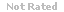Loading... Please wait...

(TCOs 4, 8) Assume you are given the following If structure. Assume that X = 20, Y = 10, and Z = 5. What will be the output?

Price:
\$5.99

Product Description

1. (TCOs 4, 8) Assume you are given the following If structure.
Assume that X = 20, Y = 10, and Z = 5. What will be the output?
2. (TCOs 4, 8) Assume you are given the following If structure.

Assume that R = 7, S = 7, and T = 11. What will be the output?
3. (TCOs 4, 8) Employees at a certain company get a bonus at the end of the year based on years of service. The bonus is a percentage of their annual salary determined as follows.
Less than 5 years                     2%
5 to 9 years                              4%
10 to 19 years                          7%
20 years or more                      12%
Write a pseudocode algorithm that will input the years employed and the salary, and will calculate and print the bonus rate and bonus amount. Use structured pseudocode as demonstrated in the lectures for code, ensure that all variables are declared, prompt the user for the appropriate input, and display a meaningful output message. Make sure that the years and salary are both greater than 0.

Customers Who Viewed This Product Also Viewed

• \$14.99
• \$14.99
• \$1.99
• \$19.99
• \$6.99

• \$5.99